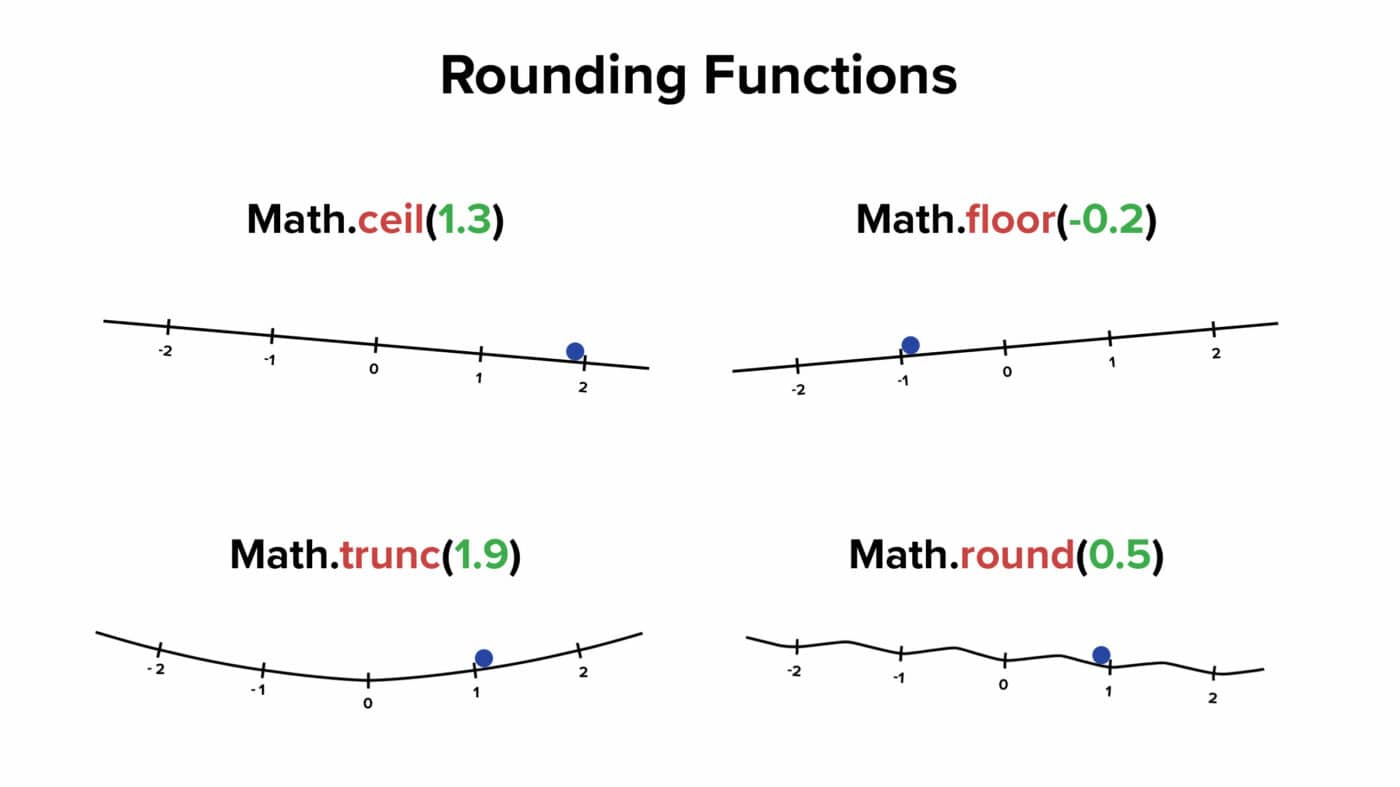# floor

Learn how to use the floor function in Notion formulas.

The `floor()` function returns the largest integer that is less than or equal to its argument.

```.wp-block-code {
border: 0;
}

.wp-block-code > div {
overflow: auto;
}

.shcb-language {
border: 0;
clip: rect(1px, 1px, 1px, 1px);
-webkit-clip-path: inset(50%);
clip-path: inset(50%);
height: 1px;
margin: -1px;
overflow: hidden;
position: absolute;
width: 1px;
word-wrap: normal;
word-break: normal;
}

.hljs {
box-sizing: border-box;
}

.hljs.shcb-code-table {
display: table;
width: 100%;
}

.hljs.shcb-code-table > .shcb-loc {
color: inherit;
display: table-row;
width: 100%;
}

.hljs.shcb-code-table .shcb-loc > span {
display: table-cell;
}

.wp-block-code code.hljs:not(.shcb-wrap-lines) {
white-space: pre;
}

.wp-block-code code.hljs.shcb-wrap-lines {
white-space: pre-wrap;
}

.hljs.shcb-line-numbers {
border-spacing: 0;
counter-reset: line;
}

.hljs.shcb-line-numbers > .shcb-loc {
counter-increment: line;
}

.hljs.shcb-line-numbers .shcb-loc > span {
}

.hljs.shcb-line-numbers .shcb-loc::before {
border-right: 1px solid #ddd;
content: counter(line);
display: table-cell;
text-align: right;
-webkit-user-select: none;
-moz-user-select: none;
-ms-user-select: none;
user-select: none;
white-space: nowrap;
width: 1%;
}```floor(number)

number.floor()
``````

In other words, `floor()` rounds a non-whole number to the next smallest integer. When used on an integer, it will return that integer.

``````floor(4.2) /* Output: 4 */

3.845.floor() /* Output: 3 */

floor(4) /* Output: 4 */
``````

It’s worth noting that `floor()` rounds “downward towards negative infinity”, while its counterpart, ceil, rounds “upward towards positive infinity”Note: This graphic shows JavaScript rounding functions. Notion formulas don’t have a trunc() function, but you can create one using ternary syntax (shown below).

When a negative value is passed as `floor()`‘s argument, you’ll still get a smaller value.

``````floor(-3.7) /* Output: -4 */
``````

`floor()` does not round “towards zero”, and ceil does not round “away from zero”.

In JavaScript, the Math.trunc function rounds “towards” zero. It simply removes all decimal values from a non-whole number – e.g. `Math.trunc(3.7) == 3` and `Math.trunc(-3.7) == -3`.

Notion does not have a `trunc()` function, but you can create one using an if-then statement:

``````/* Assume a number property exists called Num */
prop("Num") >= 0 ? prop("Num").floor() : prop("Num").ceil()
``````

As stated above, Notion does not currently provide a `trunc()` function.

The example database below provides a working example of a `trunc()` polyfill (a.k.a. custom code that replicates the functionality of a missing function), which uses both `floor()` and ceil.

Floor and ceiling values are also provided for reference.

``````/* Written with ternary operator (? and :) */
prop("Num") >= 0
? floor(prop("Num"))
: ceil(prop("Num"))

/* Written with if() */
if(
prop("Num") >= 0,
floor(
prop("Num")
),
ceil(
prop("Num")
)
)
``````

A proper `trunc()` function (which does not exist in Notion) would simply chop all of the digits after the decimal off of a number and return the integer.

To create an effective polyfill for this function, we must first determine if our input number is positive or negative. We do this with `prop("Num") >= 0`, though the same could be accomplished with the sign function: `sign(prop("Num")) == 1`

If the input number is positive, we pass it as an argument to `floor()`.

If it is negative, we must instead pass it as an argument to ceil.

You can check this in the example table above by comparing the output in the Trunc column to that of the Floor and Ceil columns.